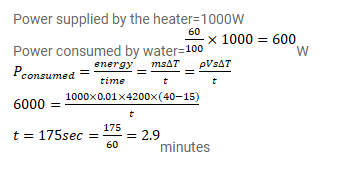# An immersion heater rated 1000W,Question:

An immersion heater rated $1000 \mathrm{~W}, 220 \mathrm{~V}$ is used to heat $0.01 \mathrm{~m}^{3}$ of water. Assuming that the power is supplied at $220 \mathrm{~V}$ and $60 \%$ of the power supplied is used to heat the water, how long will it take to increase the temperature of the water from $15^{\circ} \mathrm{C}$ to $40^{\circ} \mathrm{C}_{?}$

Solution: